# Fraction Calc - Fraction Calculator

## Fraction Calc computes mixed number improper and proper fractions.

4.33 EDUCATION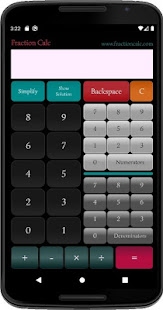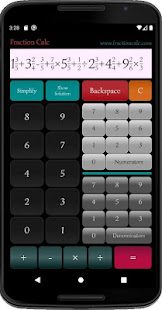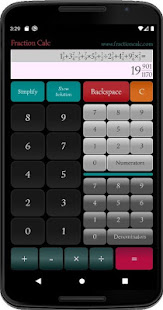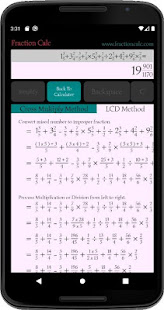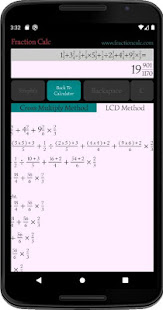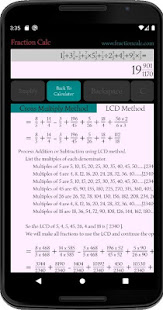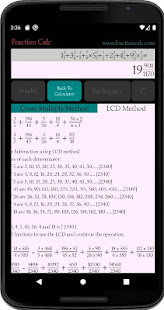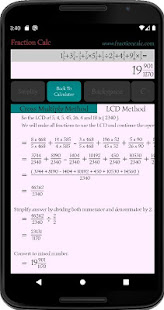Fraction Calc is a fraction calculator app designed to compute from basic to complex calculation such as the combination of mixed number, improper, and proper fraction.
It is referred as mixed fraction calculator that can calculate 3 or more fractions at one time.

Mixed fraction calculator is intended for students who want to improve their computing skills and for teachers who want to double check their solutions.
Fraction Calc provides exact solutions to check if the result of a certain operation is correct by showing the step by step solution. With this method, the margin of error is marginalized.

Fraction Calc was designed to help the students to speed up their learning process by providing the standard process on how the calculation is being done.
Fraction Calc helps those businesses, employees, and individuals whose work is related or involved a lot of fractions computation.

Fraction Calc is a mixed fraction calculator that processes the calculations according to MDAS rules. It means that multiplication and division has a higher precedence than addition and subtraction.

For the operation with the same precedence like division and multiplication or addition and subtraction, the rule is that the computation should be processed from LEFT to RIGHT.

For operation involving whole numbers, they have to be converted first to improper fraction before computation is being made. This is the standard process that has been followed since the dawn of mathematics.

How the Calculation is Done?
1.) If all inputs are whole numbers, Fraction Calc behaves like normal calculator except when there is a division operation.
2.) Convert whole numbers, if there is any, to improper fractions.
3.) Check for Multiplication or Division and calculate it from Left to Right according the rules of multiplying and dividing of fractions.
4.) Check if denominators are different and makes them the same using Cross-Multiply or LCD Method.
5.) If all denominators are equal, addition or subtraction is done according to the rules.
6.) The result is simplified if it is not in its simplest form.
7.) The result is converted to mixed number if numerator is bigger than denominator. If both are equal, they are converted to whole number.

Only one fraction can be simplified at a time.

In version 7.0, we are removing '=' in solution display where there is no number after it. The problem can be reproduced using this equation "6 times 4 divided by 1/4". In version 8.0, we adding the "Version 8.0" label on the title. In version 9.0, we are adding the step by step solution of simplifying fraction to its simplest form.

### Details and Recent Ratings

Size: 0 MB
Version: 9.0 by
Updated: 28 January 2020 (1107 days ago)
Released:
Installations: more than 10 000
5 Stars: 98
4 Stars: 0
3 Stars: 14
2 Stars: 0
1 Star: 14

### Similar apps

Android Game Categories
Android App Categories